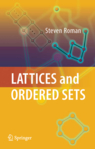#Lattices and Ordered Sets

This book is intended to be a thorough introduction to the subject of order and lattices, with an emphasis on the latter. It can be used for a course at the graduate or advanced undergraduate level or for independent study. Prerequisites are kept to a minimum, but an introductory course in abstract algebra is highly recommended, since many of the examples are drawn from this area. This is a book on pure mathematics: I do not discuss the applications of lattice theory to physics, computer science or other disciplines.

Lattice theory began in the early 1890s, when Richard Dedekind wanted to know the answer to the following question: Given three subgroups A, B and C of an abelian group G, what is the largest number of distinct subgroups that can be formed using these subgroups and the operations of intersection and sum (join)? In lattice-theoretic terms, this is the number of elements in the relatively free modular lattice on three generators. Dedekind answered this question (the answer is ) and wrote two papers on the subject of lattice theory, but then the subject lay relatively dormant until Garrett Birkhoff, Oystein Ore and others picked it up in the 1930s. Since then, many noted mathematicians have contributed to the subject, including Garrett Birkhoff, Richard Dedekind, Israel Gelfand, George Grätzer, Aleksandr Kurosh, Anatoly Malcev, Oystein Ore, Gian-Carlo Rota, Alfred Tarski and Johnny von Neumann.

This book is divided into two parts. The first part (Chapters 1–9) outlines the basic notions of the theory and the second part (Chapters 10–12) contains independent topics.

Specifically, Chapter 1 describes the basic theory of partially ordered sets, including duality, chain conditions and Dilworth's theorem. Chapter 2 is devoted to the basics of well-ordered sets, especially ordinal and cardinal numbers, a topic that is usually presented only in classes on set theory. However, I have included it here for two reasons. First, we will have a few occasions to use ordinal numbers, most notably in describing conditions that characterize the incompleteness of a lattice and in the discussion of fixed points. Second, it is my experience that many students of algebra do not take a class in set theory and so this seems a reasonable place to get a first exposure to this important subject. In any case, the chapter may be omitted upon first reading without severe consequences: Only a few theorems will be inaccessible to readers with no background in the subject. However, I have also included nonordinal versions (for countable lattices) of these theorems.

Chapter 3 begins the study of lattices, introducing the key properties such as join-irreducibility, completeness, denseness, lattice homomorphisms, the -down map, ideals and filters, prime and maximal ideals and the Dedekind-MacNeille completion.

Chapter 4 is devoted to the basics of modular and distributive lattices, including the many characterizations of these important types of lattices and the effect of distributivity on join-irreducibility and prime ideals.

Chapter 5 is devoted to Boolean algebras. This is a vast field, with many applications, but we concentrate on the lattice-theoretic concepts, such as characterizing Boolean algebras as Boolean rings, or in terms of weak forms of modularity or de Morgan's laws. We also discuss complete and infinite distributivity.

Chapter 6 concerns the representation theory of distributive lattices. We describe the representation of a distributive lattice with the DCC as a power set sublattice, the representation of complete atomic Boolean algebras as power sets and the representation of an arbitrary distributive lattice as a sublattice of down-sets of prime ideals.

Chapter 7 is devoted to algebraic lattices, a topic that should be of special interest to all those interested in abstract algebra. Here we prove that a lattice is algebraic if and only if it is isomorphic to the subalgebra lattice of some algebra.

Chapter 8 concerns the existence of maximal and prime ideals in lattices. Here we discuss various types of separation theorems and their relationship with distributivity, including the famous Boolean prime ideal theorem. This material is key to an understanding of the Stone and Priestley duality theory, described in a later chapter. Chapter 9 is devoted to congruence relations on lattices, quotient lattices, standard congruences and sectionally complemented lattices.

Chapters 10–12 are topics chapters. They can be covered in any order. Chapter 10 is devoted to the duality theory for bounded distributive lattices and Boolean algebras. We cover the finite case first, where topological notions are not required. Then we discuss the necessary topics concerning Boolean and ordered Boolean spaces. Although very little point-set topology is used, a brief appendix is included for readers who are not familiar with the subject. In both the finite and nonfinite cases, the dualities are most elegantly described using the language of category theory. For readers who are not familiar with this language, an appendix is provided with just enough category theory for our discussion in the text. (It is not so much the results of category theory that we need, but rather the language of category theory.)

Chapter 11 is an introduction to free lattices and Chapter 12 covers fixed-point theorems for monotone and for inflationary functions on complete partially ordered sets and complete lattices.

2008, ISBN 0-387-78900-2, 305 pp., Hardcover

Preface.
Partially Ordered Sets.
Well-Ordered Sets.
Lattices.
Modular and Distributive Lattices.
Boolean Algebras.
The Representation of Distributive Lattices.
Algebraic Lattices.
Prime and Maximal Ideals: Separation Theorems.
Congruence Relations on Lattices.
Duality for Distributive Lattices: The Priestley Topology.
Free Lattices.
Fixed-Point Theorems.
Appendix A: A Bit of Topology.
Appendix B: A Bit of Category Theory.
References.
Index of Symbols.
Index.